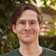# Leetcode - Episode 15 - Three Tree Qs (3 x E)

January 15, 2019

I felt like it was time to knock some of these tree questions out. I find that solving tree questions in Python leads to simple, elegant code.

I went over Heap’s Algorithm for solving permutation problems earlier. It hasn’t fully clicked with me yet though. More studying is required — as well as checking out alternative solutions. Heap’s feels a little heavy-handed to me.

### 226. Invert Binary Tree

Problem: Invert a binary tree.

I like the check I use here before I invert — `if node.left or node.right:`. It’s instantly obvious that it will work for all three siutations, two leafs, one leaf, None.

``````# Definition for a binary tree node.
# class TreeNode(object):
#     def __init__(self, x):
#         self.val = x
#         self.left = None
#         self.right = None

class Solution(object):
def invertTree(self, root):
"""
:type root: TreeNode
:rtype: TreeNode
"""
def recurse(node):
if node.left or node.right:
node.left, node.right = node.right, node.left
if node.left:
recurse(node.left)
if node.right:
recurse(node.right)
return

if root:
recurse(root)
return root``````

This problem is a little bit of a meme. I expected it to be harder than it was but I have been revising tree algorithms recently..

Runtime complexity: `O(n)`.

Spacetime complexity: `O(n)`.

### 590. N-ary Tree Postorder Traversal

Problem: Return the postorder traversal of a tree’s values.

To think about this in another way, return the values as you would read the tree from the bottom up line by line.

``````"""
# Definition for a Node.
class Node(object):
def __init__(self, val, children):
self.val = val
self.children = children
"""
class Solution(object):
def postorder(self, root):
"""
:type root: Node
:rtype: List[int]
"""
vals = []
queue = []
if root:
queue.append(root)
while queue:
cur = queue.pop()
vals.append(cur.val)
queue.extend(cur.children)

return vals[::-1]``````

I recently learned (or, confirmed) that Python doesn’t create new memory when slicing or creating an iterator, e.g. `[1,2][::-1]` / `reversed([1,2])`. It seems obvious now that I think about it.

Runtime complexity: `O(n)`.

Space complexity: `O(n)`.

### 589. N-ary Tree Preorder Traversal

Problem: Return the preorder traversal of a tree’s values.

Another way to think about preorder traversal is Depth-First-Search order.

``````"""
# Definition for a Node.
class Node(object):
def __init__(self, val, children):
self.val = val
self.children = children
"""
class Solution(object):
def preorder(self, root):
"""
:type root: Node
:rtype: List[int]
"""
dfs_order = []

def recurse(node):
dfs_order.append(node.val)
if node.children is not None:
for i in range(0, len(node.children)):
recurse(node.children[i])
if root:
recurse(root)

return dfs_order``````

I think this is a fairly terse but understandable solution but you can only make DFS so complicated.

Runtime complexity: `O(n)`.

Spacetime complexity: `O(n)`.